Theory and Modern Applications

# A coupled system of fractional differential equations with nonlocal integral boundary conditions

## Abstract

In this paper, we prove the existence and uniqueness of solutions for a system of fractional differential equations with Riemann-Liouville integral boundary conditions of different order. Our results are based on the nonlinear alternative of Leray-Schauder type and Banach’s fixed-point theorem. An illustrative example is also presented.

MSC:34A08, 34A12, 34B15.

## 1 Introduction

In this paper, we investigate a boundary value problem of first-order fractional differential equations with Riemann-Liouville integral boundary conditions of different order given by

$\left\{\begin{array}{c}{}^{c}D_{0+}^{\alpha }u\left(t\right)=f\left(t,u\left(t\right),v\left(t\right)\right),\phantom{\rule{1em}{0ex}}t\in \left[0,1\right],\hfill \\ {}^{c}D_{0+}^{\beta }v\left(t\right)=g\left(t,u\left(t\right),v\left(t\right)\right),\phantom{\rule{1em}{0ex}}t\in \left[0,1\right],\hfill \\ u\left(0\right)=\gamma {I}^{p}u\left(\eta \right)=\gamma {\int }_{0}^{\eta }\frac{{\left(\eta -s\right)}^{p-1}}{\mathrm{\Gamma }\left(p\right)}u\left(s\right)\phantom{\rule{0.2em}{0ex}}ds,\phantom{\rule{1em}{0ex}}0<\eta <1,\hfill \\ v\left(0\right)=\delta {I}^{q}v\left(\zeta \right)=\delta {\int }_{0}^{\zeta }\frac{{\left(\zeta -s\right)}^{q-1}}{\mathrm{\Gamma }\left(q\right)}v\left(s\right)\phantom{\rule{0.2em}{0ex}}ds,\phantom{\rule{1em}{0ex}}0<\zeta <1,\hfill \end{array}$
(1.1)

where ${}^{c}D_{0+}^{\alpha }$, ${}^{c}D_{0+}^{\beta }$ denote the Caputo fractional derivatives, $0<\alpha ,\beta \le 1$, $f,g\in C\left(\left[0,1\right]×{\mathbb{R}}^{2},\mathbb{R}\right)$, and $p,q,\gamma ,\delta \in \mathbb{R}$.

Fractional differential equations have recently been addressed by several researchers for a variety of problems. Fractional differential equations arise in many engineering and scientific disciplines as the mathematical modeling of systems and processes in the fields of physics, chemistry, aerodynamics, electrodynamics of complex medium, polymer rheology, economics, control theory, signal and image processing, biophysics, blood flow phenomena, etc. . Fractional-order differential equations are also regarded as a better tool for the description of hereditary properties of various materials and processes than the corresponding integer order differential equations. With this advantage, fractional-order models become more realistic and practical than the classical integer-order models, in which such effects are not taken into account. For some recent development on the topic, see , and the references therein. The study of a coupled system of fractional order is also very significant because this kind of system can often occur in applications. The reader is referred to the papers , and the references cited therein.

This paper is organized as follows: In Sect. 2, we present some basic materials needed to prove our main results. In Sect. 3, we prove the existence and uniqueness of solutions for the system (1.1) by applying some standard fixed-point principles.

## 2 Preliminaries

Let us introduce the space $X=\left\{u\left(t\right)|u\left(t\right)\in {C}^{1}\left(\left[0,1\right]\right)\right\}$ endowed with the norm $\parallel u\parallel =max\left\{|u\left(t\right)|,t\in \left[0,1\right]\right\}$. Obviously, $\left(X,\parallel \cdot \parallel \right)$ is a Banach space. Also, let $Y=\left\{v\left(t\right)|v\left(t\right)\in {C}^{1}\left(\left[0,1\right]\right)\right\}$ endowed with the norm $\parallel v\parallel =max\left\{|v\left(t\right)|,t\in \left[0,1\right]\right\}$. The product space $\left(X×Y,\parallel \left(u,v\right)\parallel \right)$ is also a Banach space with norm $\parallel \left(u,v\right)\parallel =\parallel u\parallel +\parallel v\parallel$.

For the convenience of the readers, we now present some useful definitions and fundamental facts of fractional calculus [1, 4].

Definition 2.1 For at least n-times continuously differentiable function $g:\left[0,\mathrm{\infty }\right)\to \mathbb{R}$, the Caputo derivative of fractional order q is defined as

${}^{c}D^{q}g\left(t\right)=\frac{1}{\mathrm{\Gamma }\left(n-q\right)}{\int }_{0}^{t}{\left(t-s\right)}^{n-q-1}{g}^{\left(n\right)}\left(s\right)\phantom{\rule{0.2em}{0ex}}ds,\phantom{\rule{1em}{0ex}}n-1

where $\left[q\right]$ denotes the integer part of the real number q.

Definition 2.2 The Riemann-Liouville fractional integral of order q is defined as

${I}^{q}g\left(t\right)=\frac{1}{\mathrm{\Gamma }\left(q\right)}{\int }_{0}^{t}\frac{g\left(s\right)}{{\left(t-s\right)}^{1-q}}\phantom{\rule{0.2em}{0ex}}ds,\phantom{\rule{1em}{0ex}}q>0,$

provided the integral exists.

The following lemmas gives some properties of Riemann-Liouville fractional integrals and Caputo fractional derivative .

Lemma 2.3 Let $p,q\ge 0$, $f\in {L}_{1}\left[a,b\right]$. Then ${I}^{p}{I}^{q}f\left(t\right)={I}^{p+q}f\left(t\right)$ and ${}^{c}D^{q}{I}^{q}f\left(t\right)=f\left(t\right)$, for all $t\in \left[a,b\right]$.

Lemma 2.4 Let $\beta >\alpha >0$, $f\in {L}_{1}\left[a,b\right]$. Then ${}^{c}D^{\alpha }{I}^{\beta }f\left(t\right)={I}^{\beta -\alpha }f\left(t\right)$, for all $t\in \left[a,b\right]$.

To define the solution of the boundary value problem (1.1), we need the following lemma, which deals with a linear variant of the problem (1.1).

Lemma 2.5 Let $\gamma \ne \frac{\mathrm{\Gamma }\left(p+1\right)}{{\eta }^{p}}$. Then for a given $g\in C\left(\left[0,1\right],\mathbb{R}\right)$, the solution of the fractional differential equation

${}^{c}D^{\alpha }x\left(t\right)=g\left(t\right),\phantom{\rule{1em}{0ex}}0<\alpha \le 1$
(2.1)

subject to the boundary condition

$x\left(0\right)=\gamma {I}^{p}x\left(\eta \right)=\gamma {\int }_{0}^{\eta }\frac{{\left(\eta -s\right)}^{p-1}}{\mathrm{\Gamma }\left(p\right)}x\left(s\right)\phantom{\rule{0.2em}{0ex}}ds,\phantom{\rule{1em}{0ex}}0<\eta <1$
(2.2)

is given by

$\begin{array}{rcl}x\left(t\right)& =& \frac{1}{\mathrm{\Gamma }\left(\alpha \right)}{\int }_{0}^{t}{\left(t-s\right)}^{\alpha -1}g\left(s\right)\phantom{\rule{0.2em}{0ex}}ds\\ +\frac{\gamma \mathrm{\Gamma }\left(p+1\right)}{\mathrm{\Gamma }\left(p+1\right)-\gamma {\eta }^{p}}{\int }_{0}^{\eta }\frac{{\left(\eta -s\right)}^{p+\alpha -1}}{\mathrm{\Gamma }\left(p+\alpha \right)}g\left(s\right)\phantom{\rule{0.2em}{0ex}}ds,\phantom{\rule{1em}{0ex}}t\in \left[0,1\right].\end{array}$
(2.3)

Proof For some constant ${c}_{0}\in \mathbb{R}$, we have 

$x\left(t\right)={\int }_{0}^{t}\frac{{\left(t-s\right)}^{\alpha -1}}{\mathrm{\Gamma }\left(\alpha \right)}g\left(s\right)\phantom{\rule{0.2em}{0ex}}ds-{c}_{0}.$
(2.4)

Using the Riemann-Liouville integral of order p for (2.4), we have

$\begin{array}{rcl}{I}^{p}x\left(t\right)& =& {\int }_{0}^{t}\frac{{\left(t-s\right)}^{p-1}}{\mathrm{\Gamma }\left(p\right)}\left[{\int }_{0}^{s}\frac{{\left(s-r\right)}^{\alpha -1}}{\mathrm{\Gamma }\left(\alpha \right)}g\left(r\right)\phantom{\rule{0.2em}{0ex}}dr-{c}_{0}\right]\phantom{\rule{0.2em}{0ex}}ds\\ =& {I}^{p}{I}^{\alpha }g\left(t\right)-{c}_{0}\frac{{t}^{p}}{\mathrm{\Gamma }\left(p+1\right)}={I}^{p+\alpha }g\left(t\right)-{c}_{0}\frac{{t}^{p}}{\mathrm{\Gamma }\left(p+1\right)},\end{array}$

where we have used Lemma 2.3. Using the condition (2.2) in the above expression, we get

${c}_{0}=-\frac{\gamma \mathrm{\Gamma }\left(p+1\right)}{\mathrm{\Gamma }\left(p+1\right)-\gamma {\eta }^{p}}{I}^{p+\alpha }g\left(\eta \right).$

Substituting the value of ${c}_{0}$ in (2.4), we obtain (2.3). □

## 3 Main results

For the sake of convenience, we set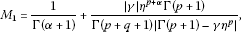(3.1)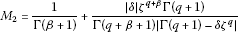(3.2)

and

${M}_{0}=min\left\{1-\left({M}_{1}{k}_{1}+{M}_{2}{\lambda }_{1}\right),1-\left({M}_{1}{k}_{2}+{M}_{2}{\lambda }_{2}\right)\right\}.$
(3.3)

Define the operator $T:X×Y\to X×Y$ by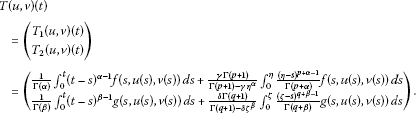The first result is based on Leray-Schauder alternative.

Lemma 3.1 (Leray-Schauder alternative,  p.4)

Let $F:E\to E$ be a completely continuous operator (i.e., a map that restricted to any bounded set in E is compact). Let

$\mathcal{E}\left(F\right)=\left\{x\in E:x=\lambda F\left(x\right)\phantom{\rule{0.25em}{0ex}}\mathit{\text{for some}}\phantom{\rule{0.25em}{0ex}}0<\lambda <1\right\}.$

Then either the set $\mathcal{E}\left(F\right)$ is unbounded, or F has at least one fixed point.

Theorem 3.2 Suppose that $\gamma \ne \frac{\mathrm{\Gamma }\left(p+1\right)}{{\eta }^{p}}$ and $\delta \ne \frac{\mathrm{\Gamma }\left(q+1\right)}{{\zeta }^{q}}$. Assume that there exist real constants ${k}_{i}$, ${\lambda }_{i}\ge 0$ ($i=1,2$) and ${k}_{0}>0$, ${\lambda }_{0}>0$ such that $\mathrm{\forall }{x}_{i}\in \mathbb{R}$ ($i=1,2$), we have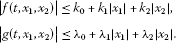In addition, it is assumed that

${M}_{1}{k}_{1}+{M}_{2}{\lambda }_{1}<1\phantom{\rule{1em}{0ex}}\mathit{\text{and}}\phantom{\rule{1em}{0ex}}{M}_{1}{k}_{2}+{M}_{2}{\lambda }_{2}<1,$

where ${M}_{1}$ and ${M}_{2}$ are given by (3.1) and (3.2), respectively. Then the boundary value problem (1.1) has at least one solution.

Proof First, we show that the operator $T:X×Y\to X×Y$ is completely continuous. By continuity of functions f and g, the operator T is continuous.

Let $\mathrm{\Omega }\subset X×Y$ be bounded. Then there exist positive constants ${L}_{1}$ and ${L}_{2}$ such that

$|f\left(t,u\left(t\right),v\left(t\right)\right)|\le {L}_{1},\phantom{\rule{2em}{0ex}}|g\left(t,u\left(t\right),v\left(t\right)\right)|\le {L}_{2},\phantom{\rule{1em}{0ex}}\mathrm{\forall }\left(u,v\right)\in \mathrm{\Omega }.$

Then for any $\left(u,v\right)\in \mathrm{\Omega }$, we have

$\begin{array}{rcl}|{T}_{1}\left(u,v\right)\left(t\right)|& \le & \frac{1}{\mathrm{\Gamma }\left(\alpha \right)}{\int }_{0}^{t}{\left(t-s\right)}^{\alpha -1}|f\left(s,u\left(s\right),v\left(s\right)\right)|\phantom{\rule{0.2em}{0ex}}ds\\ +\frac{|\gamma |\mathrm{\Gamma }\left(p+1\right)}{|\mathrm{\Gamma }\left(p+1\right)-\gamma {\eta }^{p}|}{\int }_{0}^{\eta }\frac{{\left(\eta -s\right)}^{p+\alpha -1}}{\mathrm{\Gamma }\left(p+\alpha \right)}|f\left(s,u\left(s\right),v\left(s\right)\right)|\phantom{\rule{0.2em}{0ex}}ds\\ \le & {L}_{1}\left\{\frac{1}{\mathrm{\Gamma }\left(\alpha +1\right)}+\frac{|\gamma |{\eta }^{p+\alpha }\mathrm{\Gamma }\left(p+1\right)}{\mathrm{\Gamma }\left(p+q+1\right)|\mathrm{\Gamma }\left(p+1\right)-\gamma {\eta }^{p}|}\right\}={L}_{1}{M}_{1}.\end{array}$

Similarly, we get

$\parallel {T}_{2}\left(u,v\right)\parallel \le {L}_{2}\left\{\frac{1}{\mathrm{\Gamma }\left(\beta +1\right)}+\frac{|\delta |{\zeta }^{q+\beta }\mathrm{\Gamma }\left(q+1\right)}{\mathrm{\Gamma }\left(q+\beta +1\right)|\mathrm{\Gamma }\left(q+1\right)-\delta {\zeta }^{q}|}\right\}={L}_{2}{M}_{2},$

Thus, it follows from the above inequalities that the operator T is uniformly bounded.

Next, we show that T is equicontinuous. Let $0\le {t}_{1}\le {t}_{2}\le 1$. Then we have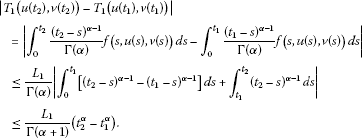Analogously, we can obtain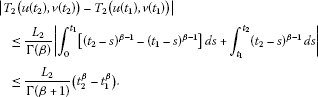Therefore, the operator $T\left(u,v\right)$ is equicontinuous, and thus the operator $T\left(u,v\right)$ is completely continuous.

Finally, it will be verified that the set $\mathcal{E}=\left\{\left(u,v\right)\in X×Y|\left(u,v\right)=\lambda T\left(u,v\right),0\le \lambda \le 1\right\}$ is bounded. Let $\left(u,v\right)\in \mathcal{E}$, then $\left(u,v\right)=\lambda T\left(u,v\right)$. For any $t\in \left[0,1\right]$, we have

$u\left(t\right)=\lambda {T}_{1}\left(u,v\right)\left(t\right),\phantom{\rule{2em}{0ex}}v\left(t\right)=\lambda {T}_{2}\left(u,v\right)\left(t\right).$

Then

$|u\left(t\right)|\le \left\{\frac{1}{\mathrm{\Gamma }\left(\alpha +1\right)}+\frac{|\gamma |{\eta }^{p+\alpha }\mathrm{\Gamma }\left(p+1\right)}{\mathrm{\Gamma }\left(p+q+1\right)|\mathrm{\Gamma }\left(p+1\right)-\gamma {\eta }^{p}|}\right\}\left({k}_{0}+{k}_{1}|u\left(t\right)|+{k}_{2}|v\left(t\right)|\right)$

and

$|v\left(t\right)|\le \left\{\frac{1}{\mathrm{\Gamma }\left(\beta +1\right)}+\frac{|\delta |{\zeta }^{q+\beta }\mathrm{\Gamma }\left(q+1\right)}{\mathrm{\Gamma }\left(q+\beta +1\right)|\mathrm{\Gamma }\left(q+1\right)-\delta {\zeta }^{q}|}\right\}\left({\lambda }_{0}+{\lambda }_{1}|u\left(t\right)|+{\lambda }_{2}|v\left(t\right)|\right).$

Hence, we have

$\parallel u\parallel \le {M}_{1}\left({k}_{0}+{k}_{1}\parallel u\parallel +{k}_{2}\parallel v\parallel \right)$

and

$\parallel v\parallel \le {M}_{2}\left({\lambda }_{0}+{\lambda }_{1}\parallel u\parallel +{\lambda }_{2}\parallel v\parallel \right),$

which imply that

$\parallel u\parallel +\parallel v\parallel =\left({M}_{1}{k}_{0}+{M}_{2}{\lambda }_{0}\right)+\left({M}_{1}{k}_{1}+{M}_{2}{\lambda }_{1}\right)\parallel u\parallel +\left({M}_{1}{k}_{2}+{M}_{2}{\lambda }_{2}\right)\parallel v\parallel .$

Consequently,

$\parallel \left(u,v\right)\parallel \le \frac{{M}_{1}{k}_{0}+{M}_{2}{\lambda }_{0}}{{M}_{0}},$

for any $t\in \left[0,1\right]$, where ${M}_{0}$ is defined by (3.3), which proves that $\mathcal{E}$ is bounded. Thus, by Lemma 3.1, the operator T has at least one fixed point. Hence, the boundary value problem (1.1) has at least one solution. The proof is complete. □

In the second result, we prove existence and uniqueness of solutions of the boundary value problem (1.1) via Banach’s contraction principle.

Theorem 3.3 Assume that $f,g:\left[0,1\right]×{\mathbb{R}}^{2}\to \mathbb{R}$ are continuous functions and there exist constants ${m}_{i}$, ${n}_{i}$, $i=1,2$ such that for all $t\in \left[0,1\right]$ and ${u}_{i},{v}_{i}\in \mathbb{R}$, $i=1,2$,

$|f\left(t,{u}_{1},{u}_{2}\right)-f\left(t,{v}_{1},{v}_{2}\right)|\le {m}_{1}|{u}_{1}-{v}_{1}|+{m}_{2}|{u}_{2}-{v}_{2}|$

and

$|g\left(t,{u}_{1},{u}_{2}\right)-g\left(t,{v}_{1},{v}_{2}\right)|\le {n}_{1}|{u}_{1}-{v}_{1}|+{n}_{2}|{u}_{2}-{v}_{2}|.$

${M}_{1}\left({m}_{1}+{m}_{2}\right)+{M}_{2}\left({n}_{1}+{n}_{2}\right)<1,$

where ${M}_{1}$ and ${M}_{2}$ are given by (3.1) and (3.2), respectively. Then the boundary value problem (1.1) has a unique solution.

Proof Define ${sup}_{t\in \left[0,1\right]}f\left(t,0,0\right)={N}_{1}<\mathrm{\infty }$ and ${sup}_{t\in \left[0,1\right]}g\left(t,0,0\right)={N}_{2}<\mathrm{\infty }$ such that

$r\ge \frac{{N}_{1}{M}_{1}+{N}_{2}{M}_{2}}{1-{M}_{1}\left({m}_{1}+{m}_{2}\right)-{M}_{2}\left({n}_{1}+{n}_{2}\right)}.$

We show that $T{B}_{r}\subset {B}_{r}$, where ${B}_{r}=\left\{\left(u,v\right)\in X×Y:\parallel \left(u,v\right)\parallel \le r\right\}$.

For $\left(u,v\right)\in {B}_{r}$, we have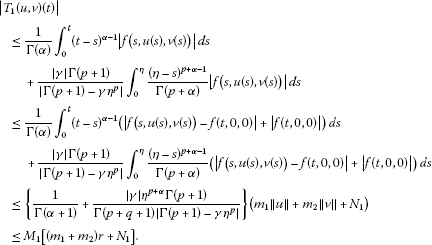Hence,

$\parallel {T}_{1}\left(u,v\right)\left(t\right)\parallel \le {M}_{1}\left[\left({m}_{1}+{m}_{2}\right)r+{N}_{1}\right].$

In the same way, we can obtain that

$\parallel {T}_{2}\left(u,v\right)\left(t\right)\parallel \le {M}_{2}\left[\left({n}_{1}+{n}_{2}\right)r+{N}_{2}\right].$

Consequently, $\parallel T\left(u,v\right)\left(t\right)\parallel \le r$.

Now for $\left({u}_{2},{v}_{2}\right),\left({u}_{1},{v}_{1}\right)\in X×Y$, and for any $t\in \left[0,1\right]$, we get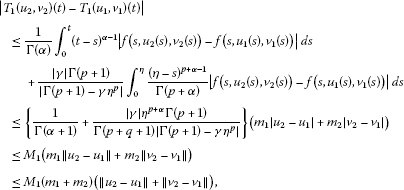and consequently we obtain

$\parallel {T}_{1}\left({u}_{2},{v}_{2}\right)\left(t\right)-{T}_{1}\left({u}_{1},{v}_{1}\right)\parallel \le {M}_{1}\left({m}_{1}+{m}_{2}\right)\left(\parallel {u}_{2}-{u}_{1}\parallel +\parallel {v}_{2}-{v}_{1}\parallel \right).$
(3.4)

Similarly,

$\parallel {T}_{2}\left({u}_{2},{v}_{2}\right)\left(t\right)-{T}_{2}\left({u}_{1},{v}_{1}\right)\parallel \le {M}_{2}\left({n}_{1}+{n}_{2}\right)\left(\parallel {u}_{2}-{u}_{1}\parallel +\parallel {v}_{2}-{v}_{1}\parallel \right).$
(3.5)

It follows from (3.4) and (3.5) that

$\parallel T\left({u}_{2},{v}_{2}\right)\left(t\right)-T\left({u}_{1},{v}_{1}\right)\left(t\right)\parallel \le \left[{M}_{1}\left({m}_{1}+{m}_{2}\right)+{M}_{2}\left({n}_{1}+{n}_{2}\right)\right]\left(\parallel {u}_{2}-{u}_{1}\parallel +\parallel {v}_{2}-{v}_{1}\parallel \right).$

Since ${M}_{1}\left({m}_{1}+{m}_{2}\right)+{M}_{2}\left({n}_{1}+{n}_{2}\right)<1$, therefore, T is a contraction operator. So, by Banach’s fixed-point theorem, the operator T has a unique fixed point, which is the unique solution of problem (1.1). This completes the proof. □

Example 3.4 Consider the following system of fractional boundary value problem:

$\left\{\begin{array}{c}{}^{c}D^{1/2}x\left(t\right)=\frac{1}{4{\left(t+2\right)}^{2}}\frac{|u\left(t\right)|}{1+|u\left(t\right)|}+1+\frac{1}{16}{sin}^{2}v\left(t\right),\phantom{\rule{1em}{0ex}}t\in \left[0,1\right],\hfill \\ {}^{c}D^{1/2}x\left(t\right)=\frac{1}{32\pi }sin\left(2\pi u\left(t\right)\right)+\frac{|v\left(t\right)|}{16\left(1+|v\left(t\right)|\right)}+\frac{1}{2},\phantom{\rule{1em}{0ex}}t\in \left[0,1\right],\hfill \\ u\left(0\right)=\sqrt{3}{I}^{3/2}u\left(\frac{1}{3}\right),\hfill \\ v\left(0\right)=\sqrt{2}{I}^{1/2}v\left(\frac{1}{2}\right).\hfill \end{array}$
(3.6)

Here, $\alpha =1/2$, $\gamma =\sqrt{3}$, $p=3/2$, $\eta =1/3$, $\beta =1/2$, $\delta =\sqrt{2}$, $q=1/2$, $\zeta =1/2$, and $f\left(t,u,v\right)=\frac{1}{4{\left(t+2\right)}^{2}}\frac{|u|}{1+|u|}+1+\frac{1}{8}{sin}^{2}v$ and $g\left(t,u,v\right)=\frac{1}{32\pi }sin\left(2\pi u\right)+\frac{|v|}{16\left(1+|v|\right)}+\frac{1}{2}$. Note that $\gamma =\sqrt{3}\ne \mathrm{\Gamma }\left(p+1\right)/{\eta }^{p}=\mathrm{\Gamma }\left(5/2\right)/{\left(1/3\right)}^{3/2}$ and $\delta =\sqrt{2}\ne \mathrm{\Gamma }\left(q+1\right)/{\zeta }^{q}=\mathrm{\Gamma }\left(3/2\right)/{\left(1/2\right)}^{1/2}$. Furthermore, $|f\left(t,{u}_{1},{u}_{2}\right)-f\left(t,{v}_{1},{v}_{2}\right)|\le \frac{1}{16}|{u}_{1}-{u}_{2}|+\frac{1}{16}|{v}_{1}-{v}_{2}|$, $|g\left(t,{u}_{1},{u}_{2}\right)-g\left(t,{v}_{1},{v}_{2}\right)|\le \frac{1}{16}|{u}_{1}-{u}_{2}|+\frac{1}{16}|{v}_{1}-{v}_{2}|$, and

$\begin{array}{rcl}{M}_{1}\left({m}_{1}+{m}_{2}\right)+{M}_{2}\left({n}_{1}+{n}_{2}\right)& =& \frac{1}{8}\left\{\frac{2}{\sqrt{\pi }}+\frac{\sqrt{3\pi }}{2\left(9\sqrt{\pi }-4\right)}\right\}+\frac{1}{16}\left\{\frac{2}{\sqrt{\pi }}+\frac{\sqrt{2\pi }}{2\left(2-\sqrt{\pi }\right)}\right\}\\ \approx & 0.712679<1.\end{array}$

Thus, all the conditions of Theorem 3.3 are satisfied and consequently, its conclusion applies to the problem (3.6).

## References

1. Kilbas AA, Srivastava HM, Trujillo JJ North-Holland Mathematics Studies 204. In Theory and Applications of Fractional Differential Equations. Elsevier, Amsterdam; 2006.

2. Lakshmikantham V, Leela S, Vasundhara Devi J: Theory of Fractional Dynamic Systems. Cambridge Academic Publishers, Cambridge; 2009.

3. Lakshmikantham V, Vatsala AS: Basic theory of fractional differential equations. Nonlinear Anal. 2008, 69(8):2677–2682. 10.1016/j.na.2007.08.042

4. Podlubny I: Fractional Differential Equations. Academic Press, San Diego; 1999.

5. Sabatier J, Agrawal OP, Machado JAT (Eds): Advances in Fractional Calculus: Theoretical Developments and Applications in Physics and Engineering. Springer, Dordrecht; 2007.

6. Ahmad B: Existence of solutions for irregular boundary value problems of nonlinear fractional differential equations. Appl. Math. Lett. 2010, 23: 390–394. 10.1016/j.aml.2009.11.004

7. Ahmad B, Nieto JJ: Existence results for a coupled system of nonlinear fractional differential equations with three-point boundary conditions. Comput. Math. Appl. 2009, 58: 1838–1843. 10.1016/j.camwa.2009.07.091

8. Ahmad B, Nieto JJ: Existence of solutions for anti-periodic boundary value problems involving fractional differential equations via Leray-Schauder degree theory. Topol. Methods Nonlinear Anal. 2010, 35: 295–304.

9. Ahmad B, Nieto JJ, Pimentel J: Some boundary value problems of fractional differential equations and inclusions. Comput. Math. Appl. 2011, 62: 1238–1250. 10.1016/j.camwa.2011.02.035

10. Ahmad B, Ntouyas SK, Alsaedi A: New existence results for nonlinear fractional differential equations with three-point integral boundary conditions. Adv. Differ. Equ. 2011., 2011: Article ID 107384

11. Ahmad B, Ntouyas SK: A four-point nonlocal integral boundary value problem for fractional differential equations of arbitrary order. Electron. J. Qual. Theory Differ. Equ. 2011., 2011: Article ID 22

12. Ahmad B, Sivasundaram S: Existence and uniqueness results for nonlinear boundary value problems of fractional differential equations with separated boundary conditions. Commun. Appl. Anal. 2009, 13: 121–228.

13. Ahmad B, Sivasundaram S: On four-point nonlocal boundary value problems of nonlinear integro-differential equations of fractional order. Appl. Math. Comput. 2010, 217: 480–487. 10.1016/j.amc.2010.05.080

14. Agarwal RP, Ahmad B: Existence theory for anti-periodic boundary value problems of fractional differential equations and inclusions. Comput. Math. Appl. 2011, 62: 1200–1214. 10.1016/j.camwa.2011.03.001

15. Baleanu D, Mustafa OG, Agarwal RP: An existence result for a superlinear fractional differential equation. Appl. Math. Lett. 2010, 23: 1129–1132. 10.1016/j.aml.2010.04.049

16. Baleanu D, Mustafa OG, Agarwal RP:On ${L}^{p}$-solutions for a class of sequential fractional differential equations. Appl. Math. Comput. 2011, 218: 2074–2081. 10.1016/j.amc.2011.07.024

17. Baleanu D, Mustafa OG, Agarwal RP:Asymptotic integration of $\left(1+\alpha \right)$-order fractional differential equations. Comput. Math. Appl. 2011, 62: 1492–1500. 10.1016/j.camwa.2011.03.021

18. Baleanu D, Agarwal RP, Mustafa OG, Cosulschi M: Asymptotic integration of some nonlinear differential equations with fractional time derivative. J. Phys. A, Math. Theor. 2011., 44(5): Article ID 055203

19. Ahmad B, Nieto JJ: Existence results for a coupled system of nonlinear fractional differential equations with three-point boundary conditions. Comput. Math. Appl. 2009, 58: 1838–1843. 10.1016/j.camwa.2009.07.091

20. Su X: Boundary value problem for a coupled system of nonlinear fractional differential equations. Appl. Math. Lett. 2009, 22: 64–69. 10.1016/j.aml.2008.03.001

21. Sun J, Liu Y, Liu G: Existence of solutions for fractional differential systems with antiperiodic boundary conditions. Comput. Math. Appl. 2012. doi:10.1016/j.camwa.2011.12.083

22. Wang J, Xiang H, Liu Z: Positive solution to nonzero boundary values problem for a coupled system of nonlinear fractional differential equations. Int. J. Differ. Equ. 2010., 2010: Article ID 186928

23. Granas A, Dugundji J: Fixed Point Theory. Springer, New York; 2005.

## Author information

Authors

### Corresponding author

Correspondence to Mustafa Obaid.

### Competing interests

The authors declare that they have no competing interests.

### Authors’ contributions

Each of the authors SKN and MO contributed to each part of this study equaly and read and approved the final version of the manuscript.

## Rights and permissions

Reprints and Permissions

Ntouyas, S.K., Obaid, M. A coupled system of fractional differential equations with nonlocal integral boundary conditions. Adv Differ Equ 2012, 130 (2012). https://doi.org/10.1186/1687-1847-2012-130

• Accepted:

• Published:

• DOI: https://doi.org/10.1186/1687-1847-2012-130

### Keywords

• Caputo fractional derivative
• fractional differential systems
• integral boundary conditions
• fixed-point theorems